Arithmetic - Definition with Examples

The Complete K-5 Math Learning Program Built for Your Child

• 30 Million Kids

Loved by kids and parent worldwide

• 50,000 Schools

Trusted by teachers across schools

• Comprehensive Curriculum

Aligned to Common Core

What is Arithmetic?

Arithmetic is the branch of mathematics that deals with the study of numbers using various operations on them. Basic operations of math are addition, subtraction, multiplication and division.

These operations are denoted by the given symbols.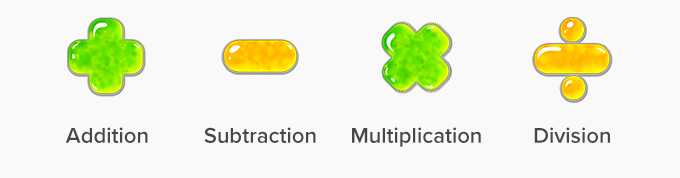• The addition is the process of taking two or more numbers and adding them together. In other words, it is the total sum of all the numbers.

• Addition of whole numbers results in a number greater than the added numbers.

Example:  If 3 kids were playing and after some time, 2 more kids join them. How many kids are there in total?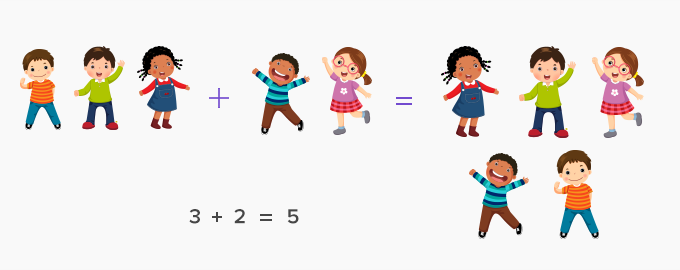This can be written as a mathematical expression as follows:

3 + 2 = 5;   Thus, 5 kids are playing in total.

Subtraction:

• Subtraction is the process in which we remove objects from the original group.

• In subtraction, the numerical value of the original number becomes less.

Example: 8 birds are sitting on a tree. After some time, 2 birds fly away. How many birds are on the tree?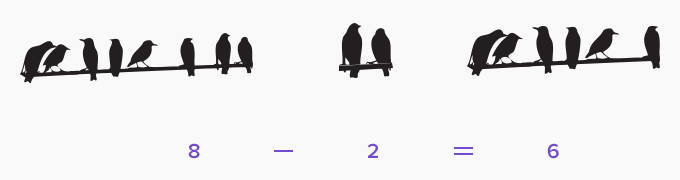8 – 2 = 6;  Hence, there are only 6 birds left on the tree.

Multiplication:

• Multiplication is adding the same number to itself a certain number of times.

• When we multiply two numbers, the result is called a product.

Example: Robin went to the garden three times and brought 5 oranges each time. How many oranges in total did Robin bring?

Solution: Robin went to the garden 3 times. Every time he brought 5 oranges.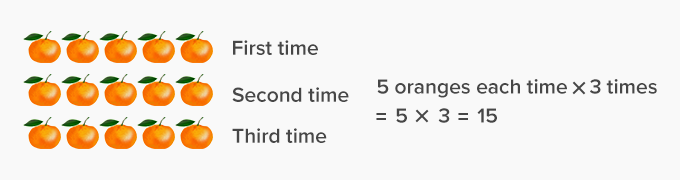This can be mathematically written as 5 × 3 = 15 oranges

Division:

• The division is breaking a large object or group into smaller parts or groups.

• The number or the larger group that gets divided is known as the dividend.

• The number that divides the dividend is known as the divisor.

• The number obtained on dividing the dividend by a divisor is called the quotient.

• The number left after dividing is called remainder.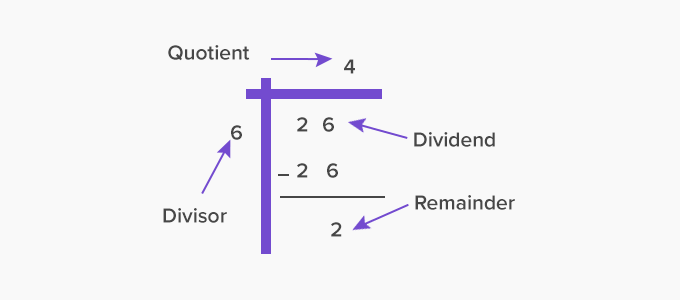Example: On distributing 26 strawberries among 6 children, each child gets 4 strawberries, and 2 strawberries are left.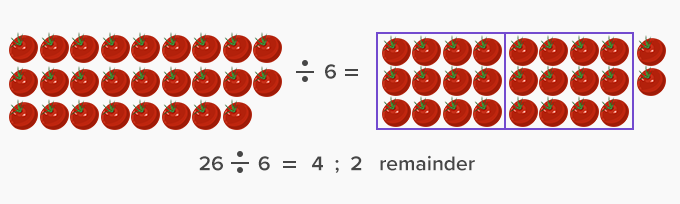Fun Facts The other branches of mathematics are Algebra, Geometry and Analysis. The word “Arithmetic” originated from Greek arithmētikē (tekhnē) which means ‘(art) of counting’, and from ‘arithmos’ which means ‘number’.
Won Numerous Awards & Honors